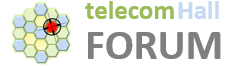# Why 64TRX (64T64R) is called 16H4V?

Hello Experts,
Why 64TRX (64T64R) is called 16H4V?
I am not able to correlate it.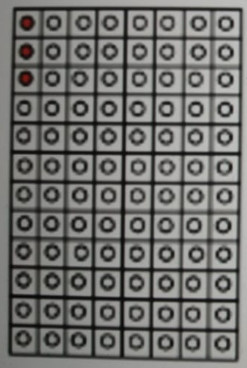I was trying to visualise it and this is what i could come up with. There are 8 column and each has 2 antenna element so total 16 in Horizontal. 4 rows.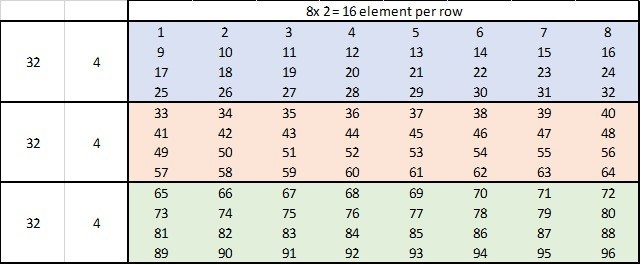There are 8 columns, so 8x2 = 16 antenna elements per column. So how it is 16H?

16 horizontal & 4 vertical.
It may be like this as shown in figure:I am also not able to visualise this.
I mean how it is 16H4V?

Each array with 3 antenna elements.
Are you are telling per array 4 rows?
Is my understanding correct?
In original figure 3 red color dots is confusing…

I have given 1 to 16 number…that is 16H. 4 diff color is 4V.
This is how 3D beamforming happen.

For your 16H and 4V what is the number of csi-rs ports you considered?

As per the diagram, 1 array = 3 elements vertically.
So, there are 4 arrays vertically.
So 4V is representing this? I don’t understand from how they got 16H.

4x8 = 32 arrays x each 3 atenna elements x each with 2 polarisation = 192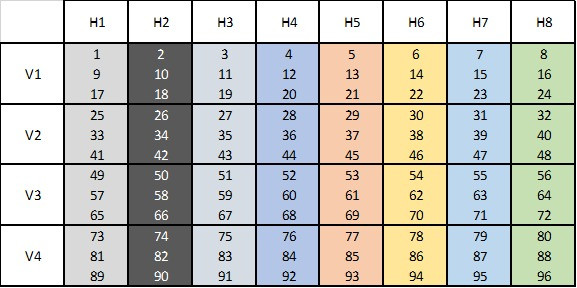I am not sure, looking difficult to accommodate in 12 csi-rs ports.

Yes, I think your understanding was corrrect.
I have changed my view.

This is really interesting.

Max number of csi-rs ports is 32.

That can be accomodated with those configs:

Which one is your case of 16H4V?

Yes, that I understood.
1 column = 4 Arrays = 4x3= 12 AE (1 array contains 3 AE).
There are 8 columns.
Total AE= 8x12 =96 AE = 96x2 pol= 192.
Main confusion is the visualization of 16H4V.

So I think 4x4 32 csi-rs ports can be used as per my understanding.So here x1…x16 is one X, so 4x4 is perfect fit in 3D beamforming.
x1 contains 12 AE so 16x12 = 192 AE.

I think 16H4V is mistake or typo.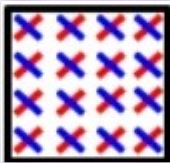Visualise my figure with this.
What is your view with this.

In that diagram, there are 12 rows and 8 columns.
How many antenna elements are being used for creating 1 horizontal beam?

All of the 12 x 8 = 96 antenna elements.